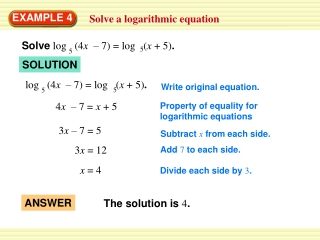DownloadDownload PresentationEXAMPLE 4

# EXAMPLE 4

Télécharger la présentation## EXAMPLE 4

- - - - - - - - - - - - - - - - - - - - - - - - - - - E N D - - - - - - - - - - - - - - - - - - - - - - - - - - -
##### Presentation Transcript

1. log (4x – 7) = log (x + 5). 5 5 Solve log (4x – 7) = log (x + 5). 5 5 ANSWER The solution is 4. EXAMPLE 4 Solve a logarithmic equation SOLUTION Write original equation. 4x – 7 = x + 5 Property of equality for logarithmic equations 3x – 7 = 5 Subtract xfrom each side. 3x = 12 Add 7 to each side. x = 4 Divide each side by 3.

2. (4x – 7) = (x – 5) ? (4 4 – 7) = (4 + 5) 9 = 9 log log log log log log 5 5 5 5 5 5 EXAMPLE 4 Solve a logarithmic equation Check: Check the solution by substituting it into the original equation. Write original equation. Substitute 4 for x. Solution checks.

3. Solve (5x – 1)= 3 (5x – 1)= 3 (5x – 1)= 3 4log4(5x – 1) = 4 log log log 4 4 b ANSWER x The solution is 13. b = x EXAMPLE 5 Exponentiate each side of an equation SOLUTION Write original equation. Exponentiate each side using base 4. 5x – 1 = 64 5x = 65 Add 1 to each side. x = 13 Divide each side by 5.

4. Check: (5x – 1) = (5 13 – 1) = 64 log log log log 4 4 4 4 3 Because 4 = 64, 64= 3. EXAMPLE 5 Exponentiate each side of an equation

5. log [2x(x – 5)] 2 10 = 10 EXAMPLE 6 Standardized Test Practice SOLUTION log 2x +log(x – 5) = 2 Write original equation. log [2x(x – 5)] = 2 Product property of logarithms Exponentiate each side using base 10. 2x(x – 5) = 100 Distributive property

6. 2 x – 5x – 50 = 0 log b 2 2x – 10x – 100 = 0 2 2x – 10x = 100 x b = x EXAMPLE 6 Standardized Test Practice Write in standard form. Divide each side by 2. (x – 10)(x + 5) = 0 Factor. x = 10 orx = – 5 Zero product property Check: Check the apparent solutions 10 and –5 using algebra or a graph. Algebra: Substitute 10 and –5 for xin the original equation.

7. log (2 10) + log (10 – 5) = 2 2 = 2 EXAMPLE 6 Standardized Test Practice log 2x + log (x – 5) = 2 log 2x+ log (x– 5) = 2 log [2(–5)] + log (–5– 5) = 2 log (–10) + log (–10) = 2 log 20 + log 5 = 2 Because log (–10) is not defined, –5 is not a solution. log 100 = 2 So, 10 is a solution.

8. ANSWER The correct answer is C. EXAMPLE 6 Standardized Test Practice Graph: Graph y = log 2x + log (x – 5) andy = 2 in the same coordinate plane. The graphs intersect only once, when x = 10. So, 10 is the only solution.

9. 10.log (x + 12) + log x =3 4 4 8.log(x – 6) = 5 2 for Examples 4, 5 and 6 GUIDED PRACTICE Solve the equation. Check for extraneous solutions. 7. ln (7x – 4) = ln (2x + 11) 9. log 5x + log (x – 1) = 2 SOLUTION 5 SOLUTION 3 4 SOLUTION SOLUTION 38